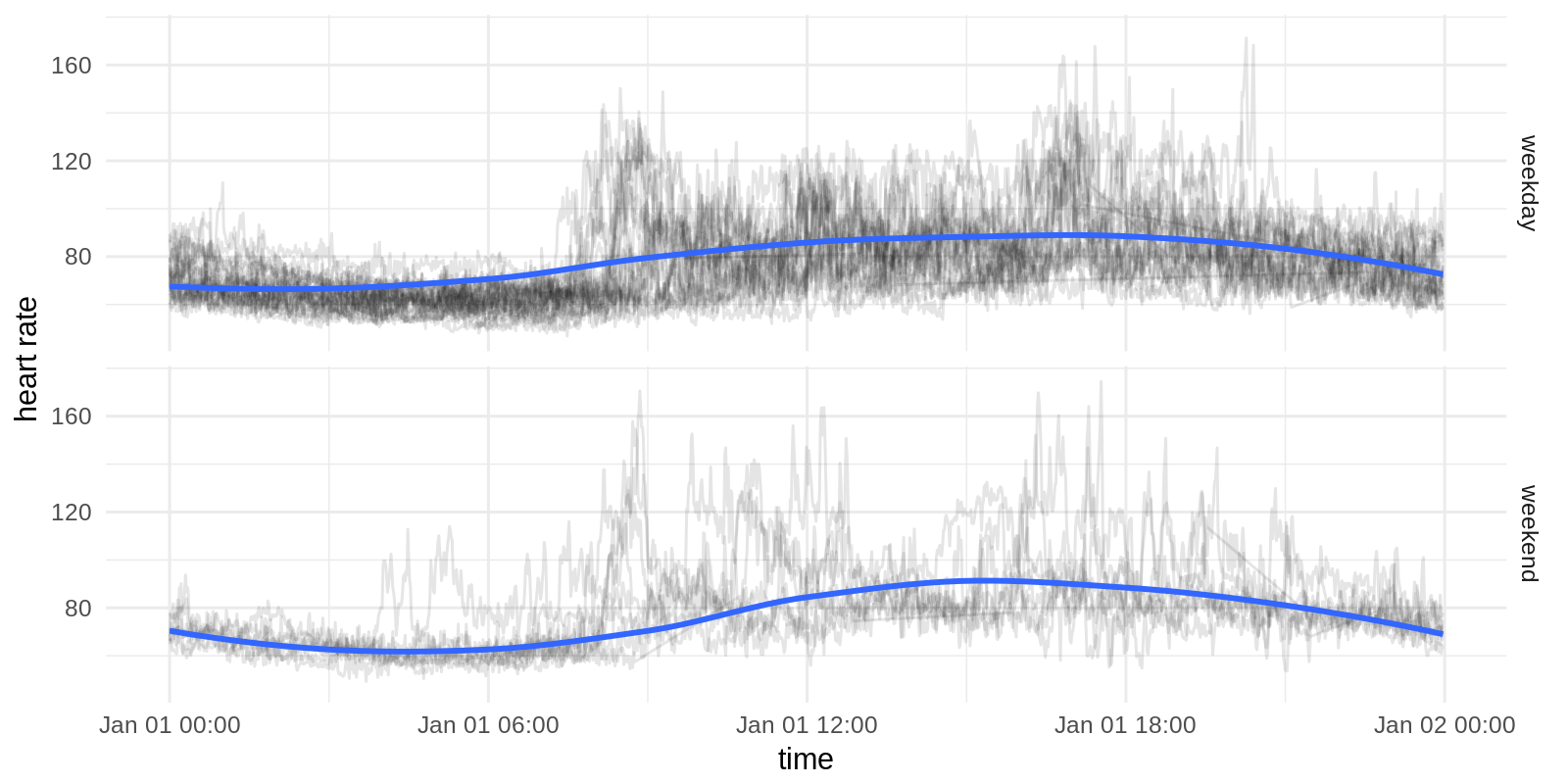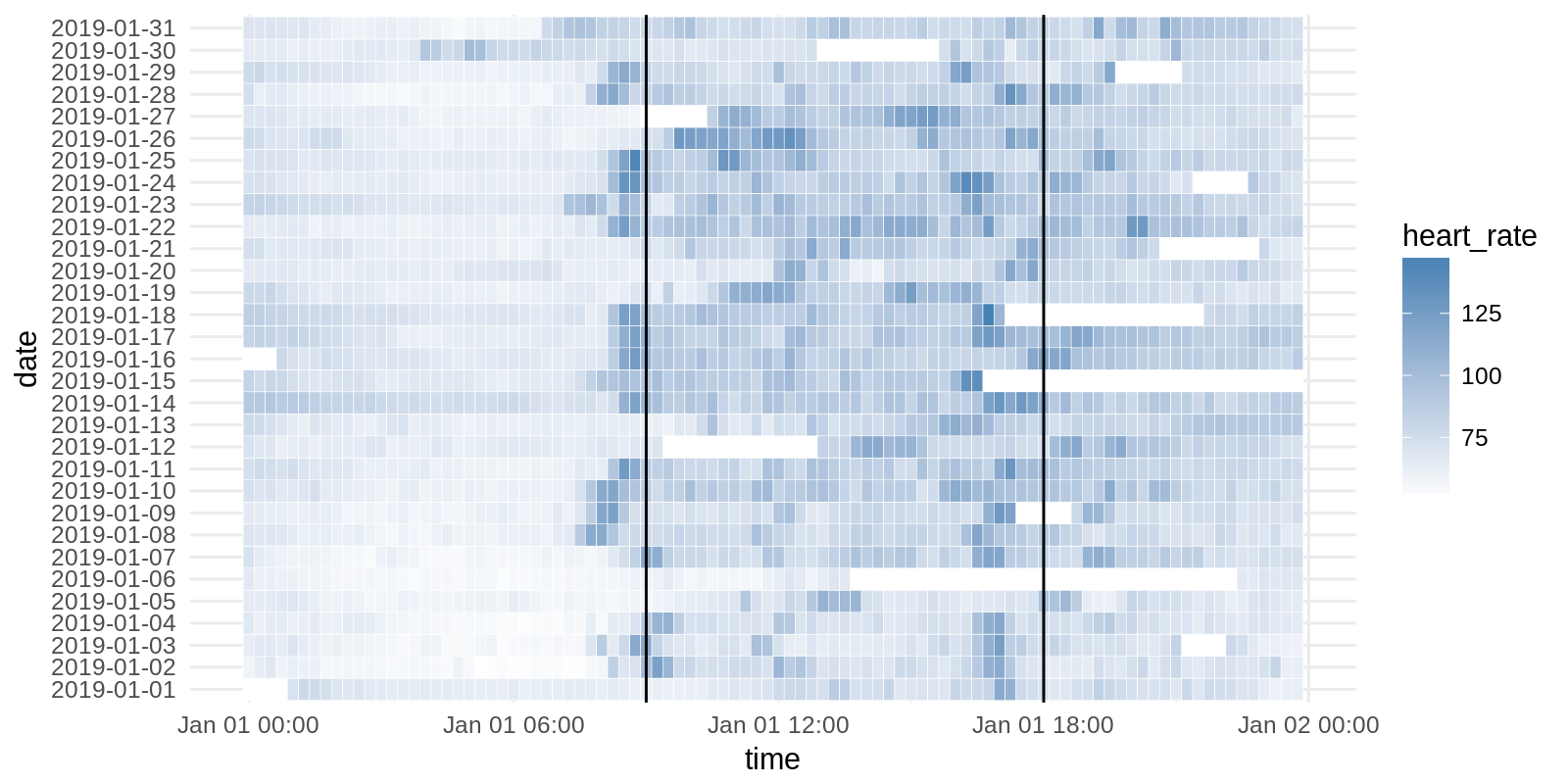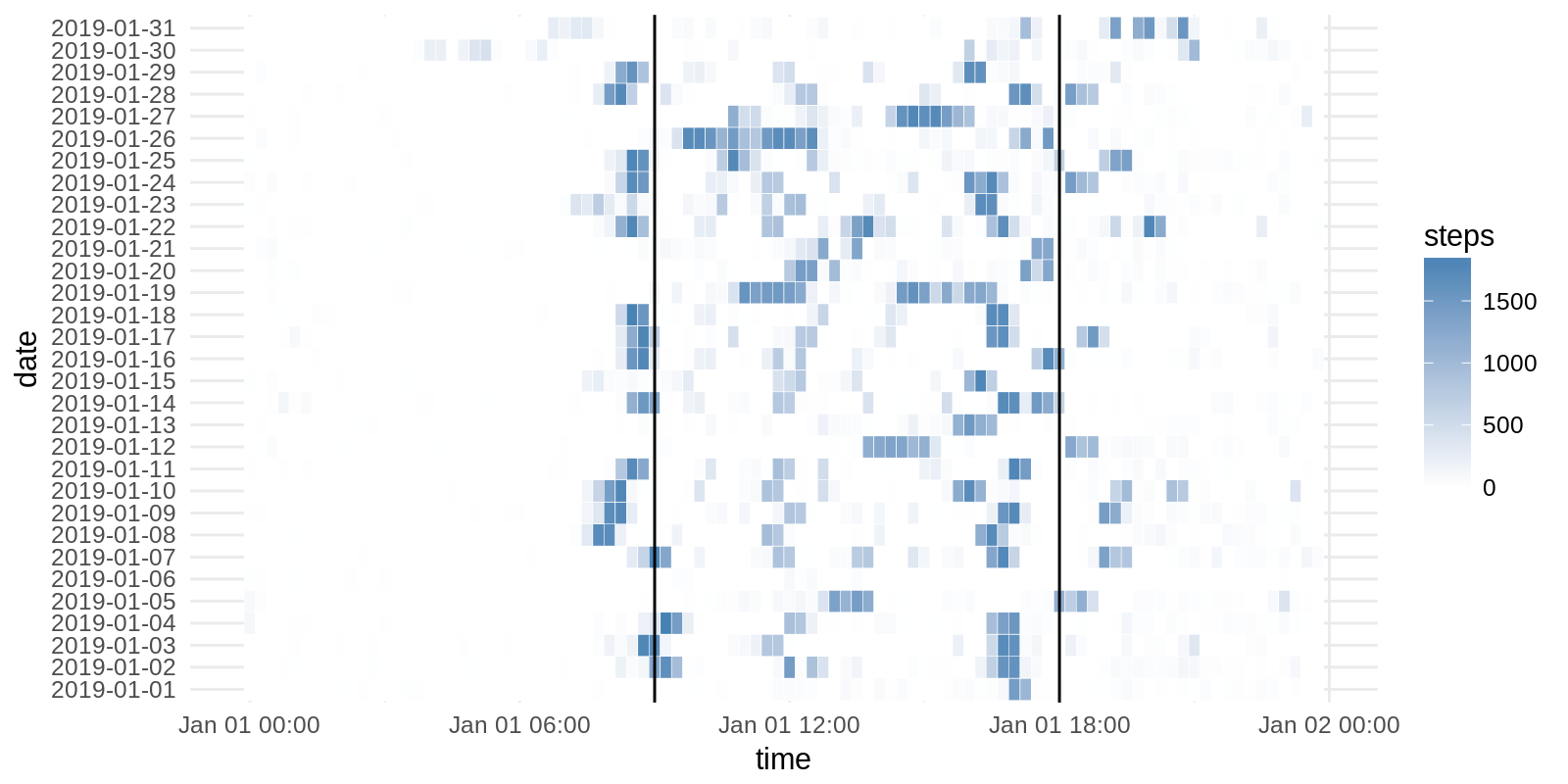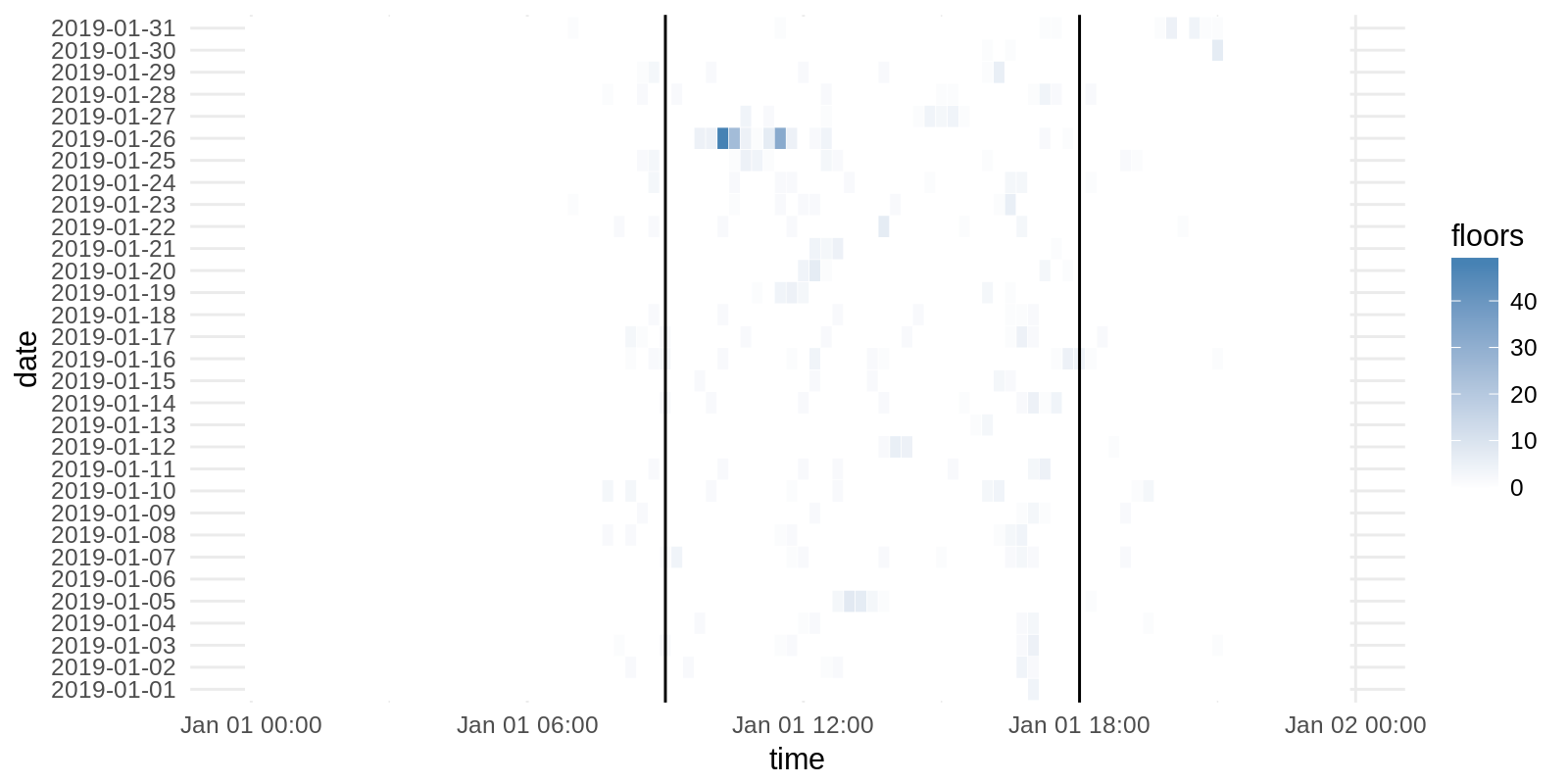# Details for `fitbit-intraday-exploration.ipynb`

#### Description

Play around with the data from the Fitbit Intraday integration.

# Analysing High-Resolution Fitbit Data¶

Bla bla

In :
```from ohapi import api
import os
import requests
import tempfile
import json
import datetime
import pandas as pd

user_details = api.exchange_oauth2_member(os.environ.get('OH_ACCESS_TOKEN'))
```

# Analyzing our heart rate over time¶

We will start by extracting our heart rate data. It is stored in Open Humans in a per-second resolution. But as this is rather noisy we will try to average it later on onto a per-minute resolution. We will focus on data from a single month.

Define the year-month below by changing the `month_of_interest` variable. The default is January 2019:

In :
```month_of_interest = '2019-01'
```
In :
```heart_rate = []
time = []
group = []
for i in user_details['data']:
if i['source'] == 'direct-sharing-191' and i['basename'] == 'fitbit-intraday-{}.json'.format(month_of_interest):
for dpoint in day['dataset']:
heart_rate.append(dpoint['value'])
time.append(datetime.datetime.strptime('2019-01-01 '+dpoint['time'][:6]+'00','%Y-%m-%d %H:%M:%S'))
group.append(day['date'])

dataframe = pd.DataFrame(
data = {
'time': time,
'heart_rate': heart_rate,
'date': group

}
)
```

Now we can load `R` and the libraries we will be using. We also pass over our first data set, which includes all the heart-rate data, collected on a per-minute basis.

In :
```%load_ext rpy2.ipython
```
In :
```%%R

library(tidyverse)
library(lubridate)
```
```/opt/conda/lib/python3.6/site-packages/rpy2/rinterface/__init__.py:186: RRuntimeWarning: Loading tidyverse: ggplot2

warnings.warn(x, RRuntimeWarning)
/opt/conda/lib/python3.6/site-packages/rpy2/rinterface/__init__.py:186: RRuntimeWarning: Conflicts with tidy packages ---------------------------------------------------

warnings.warn(x, RRuntimeWarning)
/opt/conda/lib/python3.6/site-packages/rpy2/rinterface/__init__.py:186: RRuntimeWarning: filter(): dplyr, stats
lag():    dplyr, stats

warnings.warn(x, RRuntimeWarning)
/opt/conda/lib/python3.6/site-packages/rpy2/rinterface/__init__.py:186: RRuntimeWarning:
Attaching package: ‘lubridate’

warnings.warn(x, RRuntimeWarning)
/opt/conda/lib/python3.6/site-packages/rpy2/rinterface/__init__.py:186: RRuntimeWarning: The following object is masked from ‘package:base’:

date

warnings.warn(x, RRuntimeWarning)
```

Let's now create the per-minute average heart-rates and plot them over time.

In :
```%%R -i dataframe -w 8 -h 4 --units in -r 200
aggdata <-aggregate(dataframe, by=list(dataframe\$time,dataframe\$date),
FUN=mean, na.rm=TRUE)

aggdata\$date <- as.Date(aggdata\$Group.2)
aggdata\$weekday <- wday(aggdata\$date, label=TRUE)
aggdata\$weekend <- aggdata\$weekday %in% c('Sun','Sat')

aggdata\$weekend <- ifelse(aggdata\$weekend == TRUE, "weekend", "weekday")

dataframe\$weekday <- wday(dataframe\$date, label=TRUE)
dataframe\$weekend <- dataframe\$weekday %in% c('Sun','Sat')

dataframe\$weekend <- ifelse(dataframe\$weekend == TRUE, "weekend", "weekday")

aggdata_for_smooth <-aggregate(dataframe, by=list(dataframe\$time, dataframe\$weekend),
FUN=mean, na.rm=TRUE)

aggdata_for_smooth\$weekend <- aggdata_for_smooth\$Group.2

ggplot(aggdata,aes(aggdata\$time, aggdata\$heart_rate, group=as.Date(aggdata\$Group.2))) +
geom_line(alpha=0.1) +
geom_smooth(data=aggdata_for_smooth,aes(aggdata_for_smooth\$Group.1,aggdata_for_smooth\$heart_rate,group=NULL), method='loess') +
theme_minimal() + scale_x_datetime('time') + scale_y_continuous('heart rate') + facet_grid(weekend ~ .)
```The graph shows each day as a line graph with the heart rate on the Y-axis. The blue line gives the smoothed curve observed during the day. This is rather noisy data, and there's not much to see except the lower, more uniform heart rate during sleep.

Let's see what we can learn if we sum up the data more and display it as a heat map instead of overlaying all the days. Instead of generating average heart rates per minute, we generate mean heart rates for 15 minutes intervals and plot those:

In :
```%%R -w 8 -h 4 --units in -r 200

dataframe_hr <- dataframe %>%
group_by(time = cut(time, breaks="15 min"),date=date) %>%
summarize(heart_rate = mean(heart_rate))  %>% complete(time,date) %>% as.data.frame()

dataframe_hr\$weekday <- wday(dataframe_hr\$date, label=TRUE)
dataframe_hr\$weekend <- dataframe_hr\$weekday %in% c('Sun','Sat')

dataframe_hr\$weekend <- ifelse(dataframe_hr\$weekend == TRUE, "weekend", "weekday")

ggplot(dataframe_hr, aes(as.POSIXct(time), date)) +
geom_tile(aes(fill = heart_rate), colour = "white") +
scale_fill_gradient(low = "white", high = "steelblue",na.value='white') + theme_minimal() +
scale_x_datetime('time') + geom_vline(xintercept=as.POSIXct('2019-01-01 09:00:00','%Y-%m-%d %H:%M%S')) +
geom_vline(xintercept=as.POSIXct('2019-01-01 18:00:00','%Y-%m-%d %H:%M%S'))
```The X-axis shows the time of day from midnight to midnight, on the Y-axis we see the different days for the month we have chosen. Each cell gives the average heart-rate for that interval, with white cells having a low heart rate and blue cells higher heart rates.

The two black lines show 09:00 in the morning and 18:00 (6pm). Given that I have a ~30 minute walk from home to the office and back, you can easily identify regular work days and when I made my way home/to the office.

## Step counts along the day¶

Let's now do a heatmap that does the same graph, but sums up our steps for 15 minute intervals along the day. First we extract the corresponding data from Open Humans:

In :
```steps = []
time = []
group = []
for i in user_details['data']:
if i['source'] == 'direct-sharing-191' and i['basename'] == 'fitbit-intraday-{}.json'.format(month_of_interest):
for dpoint in day['dataset']:
steps.append(dpoint['value'])
time.append(datetime.datetime.strptime('2019-01-01 '+dpoint['time'][:6]+'00','%Y-%m-%d %H:%M:%S'))
group.append(day['date'])

dataframe2 = pd.DataFrame(
data = {
'time': time,
'steps': steps,
'date': group
}
)
```

Now we can graph the data:

In :
```%%R -i dataframe2 -w 8 -h 4 --units in -r 200
dataframe3 <- dataframe2 %>%
group_by(time = cut(time, breaks="15 min"),date=date) %>%
summarize(steps = sum(steps)) %>% as.data.frame()
ggplot(dataframe3, aes(as.POSIXct(time), date)) + geom_tile(aes(fill = steps), colour = "white") +
scale_fill_gradient(low = "white", high = "steelblue") + theme_minimal() +
scale_x_datetime('time') + geom_vline(xintercept=as.POSIXct('2019-01-01 09:00:00','%Y-%m-%d %H:%M%S')) +
geom_vline(xintercept=as.POSIXct('2019-01-01 18:00:00','%Y-%m-%d %H:%M%S'))
```## Floors climbed¶

Let's now have a look at the floors climbed on different days in the same way:

In :
```floors = []
time = []
group = []
for i in user_details['data']:
if i['source'] == 'direct-sharing-191' and i['basename'] == 'fitbit-intraday-{}.json'.format(month_of_interest):
for dpoint in day['dataset']:
floors.append(dpoint['value'])
time.append(datetime.datetime.strptime('2019-01-01 '+dpoint['time'][:6]+'00','%Y-%m-%d %H:%M:%S'))
group.append(day['date'])

dataframe_floors = pd.DataFrame(
data = {
'time': time,
'floors': floors,
'date': group
}
)
```
In :
```%%R -i dataframe_floors -w 8 -h 4 --units in -r 200
dataframe_floors <- dataframe_floors %>%
group_by(time = cut(time, breaks="15 min"),date=date) %>%
summarize(floors = sum(floors)) %>% as.data.frame()
ggplot(dataframe_floors, aes(as.POSIXct(time), date)) + geom_tile(aes(fill = floors), colour = "white") +
scale_fill_gradient(low = "white", high = "steelblue") + theme_minimal() +
scale_x_datetime('time') + geom_vline(xintercept=as.POSIXct('2019-01-01 09:00:00','%Y-%m-%d %H:%M%S')) +
geom_vline(xintercept=as.POSIXct('2019-01-01 18:00:00','%Y-%m-%d %H:%M%S'))
```A single weekend outlier makes those hide very well, but there's a slight pattern in the mornings & afternoons: Getting up into the office and climbing back to Downtown Berkeley.

In [ ]:
```
```